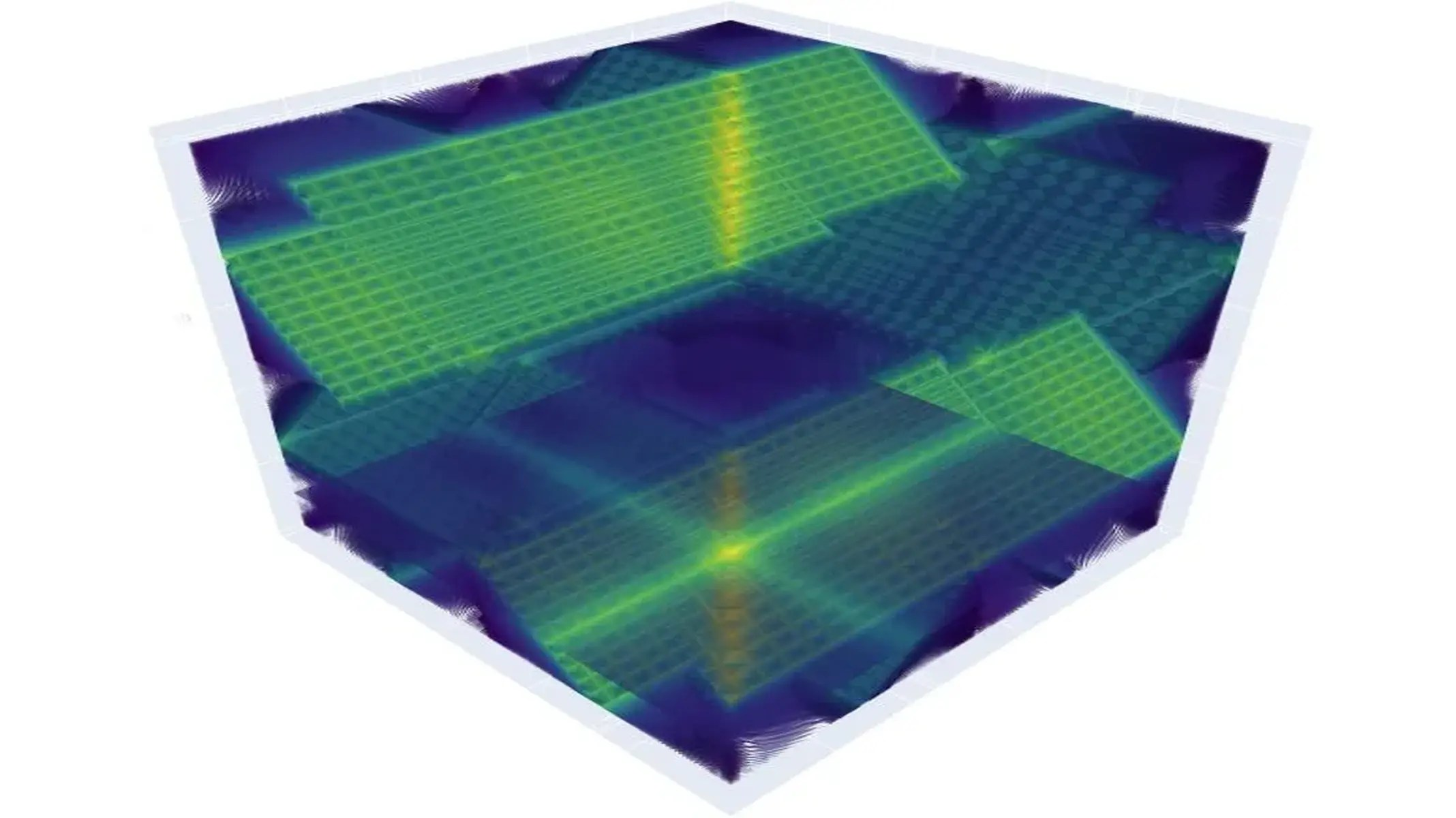# Artificial Intelligence Reduces Equation Quantum Physics Problem to Just Four EquationsArtificial Intelligence Reduces the Equation Quantum Physics Problem to Just Four Equations – Illustration of a mathematical tool used to simulate the motion and behavior of electrons on a lattice. A single interaction between two electrons is represented by each pixel. Until recently, about 100.000 equations, one for each pixel, were required to accurately capture the system. After minimizing the problem using machine learning, only four equations remained. Therefore, only four pixels will be required for a comparable visualization in the compressed version. Credit: Flatiron Institute/Domenico Di Sante